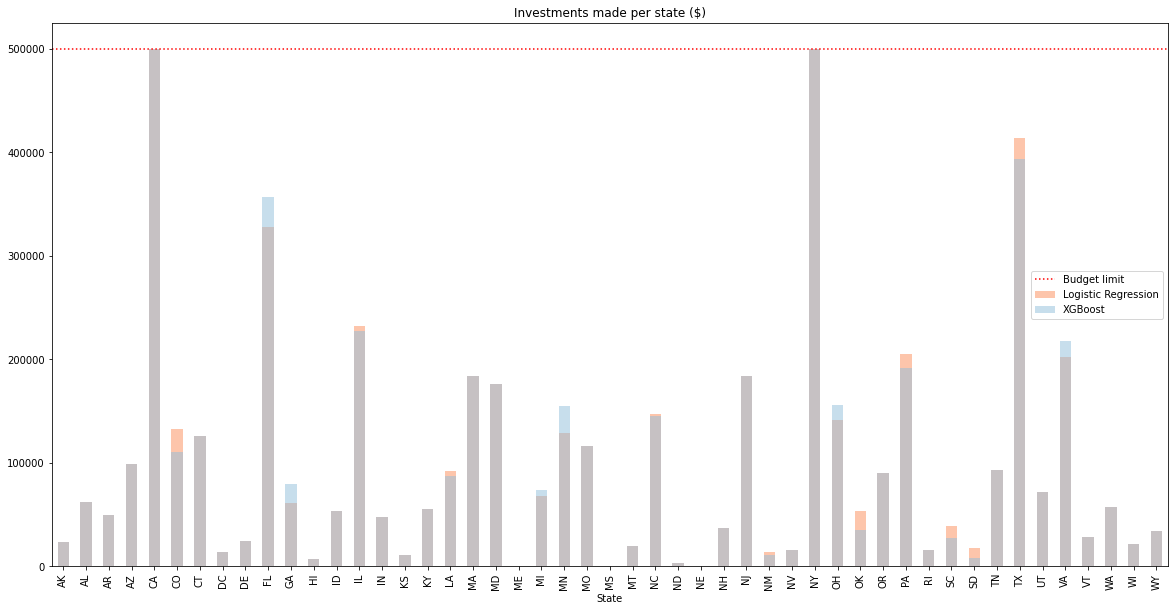# Optimization of Loan Club portfolio¶

Outside this file default probabilities were calculated for each loan in the Loan Club P2P lending service dataset. The predictions were made based on loan attributes using Logistic Regression and XGBoost. With the probability of default we calculated an expected rate of return for each loan.

This notebook optimizes a portfolio of loans with a given budget ('budget') and a limit for loan investements per state ('stateLimit').

In :
#Import necessary libraries
import gurobipy as gp
import pandas as pd
import numpy as np
import matplotlib.pyplot as plt
import itertools

In :
#Initialization
#Define constraints
budget = 5000000
stateLimit = 500000

#Create list for carrying dataframes
dfs = []

#Read data, define dataframe names and append to dfs list. More can be added.
#Logistic Reg
dataLR.name = 'Logistic Regression'
dfs.append(dataLR)

#XGBoost
dataXGB.name = 'XGBoost'
dfs.append(dataXGB)

In :
#Clean up data
def cleanup(dataframes):
#Removes redundant columns from dataframes given as arguments
cols =['Unnamed: 0','verification_status_Verified','verification_status_Source Verified','home_ownership_MORTGAGE','home_ownership_RENT','title_Debt consolidation','title_Credit card refinancing','delinq_2yrs', 'dti', 'installment', 'earliest_cr_line', 'annual_inc', 'loan_amnt', 'emp_length']
for df in dataframes:
for col in cols:
try:
df.drop(col,axis=1,inplace=True)
except KeyError:
#Column not in dataframe
pass

In :
cleanup(dfs)

In :
def optimizeModel(dataframes):
#Creates gurobi models for given Loan Club dataframes
for df in dataframes:
#Create model
m = gp.Model('portfolio')

#Create column for Gurobi variables
df['Var'] =np.NaN

# Add continous variables for each row in pandas dataframe
#Set lower boundary as 0 and upper boundary as total loan amount
for i in range(0,len(df)):
df.loc[i,'Var']= m.addVar(vtype=gp.GRB.CONTINUOUS, lb=0, ub= df.Loan[i], name="Loan{0}_from_{1}".format(i,df.State[i]))

#Update model
m.update()

#The matrix multiplication of the expected rate of return and variables optimized by gurobi
m.setObjective(df['ERR'].dot(df['Var']), gp.GRB.MAXIMIZE)

#Add 500 000 dollar state limit
#First group by state and then get the formed group
for state in df.State.unique():

#Update model
m.update()

# Optimize model to find the maximum return
m.optimize()

#Add column to data for solution value
#Gets the solution value with lambda statement
df['solution_value'] = df["Var"].apply(lambda item: item.X)
#Drop place holder for gurobi variable
df.drop(['Var'],inplace=True, axis=1)

In :
optimizeModel(dfs)

Gurobi Optimizer version 9.0.1 build v9.0.1rc0 (mac64)
Optimize a model with 50 rows, 20000 columns and 40000 nonzeros
Model fingerprint: 0xc571bd73
Coefficient statistics:
Matrix range     [1e+00, 1e+00]
Objective range  [5e+00, 3e+01]
Bounds range     [1e+03, 4e+04]
RHS range        [5e+05, 5e+06]

Concurrent LP optimizer: dual simplex and barrier
Showing barrier log only...

Presolve removed 1 rows and 1 columns
Presolve time: 0.12s
Presolved: 49 rows, 19999 columns, 39961 nonzeros

Ordering time: 0.00s

Barrier statistics:
AA' NZ     : 4.800e+01
Factor NZ  : 5.320e+02 (roughly 8 MBytes of memory)
Factor Ops : 1.049e+04 (less than 1 second per iteration)

Barrier performed 0 iterations in 0.17 seconds
Barrier solve interrupted - model solved by another algorithm

Solved with dual simplex
Solved in 4 iterations and 0.18 seconds
Optimal objective  1.495020059e+08
Gurobi Optimizer version 9.0.1 build v9.0.1rc0 (mac64)
Optimize a model with 50 rows, 20000 columns and 40000 nonzeros
Model fingerprint: 0x6055fba1
Coefficient statistics:
Matrix range     [1e+00, 1e+00]
Objective range  [5e+00, 3e+01]
Bounds range     [1e+03, 4e+04]
RHS range        [5e+05, 5e+06]

Concurrent LP optimizer: dual simplex and barrier
Showing barrier log only...

Presolve removed 1 rows and 16 columns
Presolve time: 0.15s
Presolved: 49 rows, 19984 columns, 39931 nonzeros

Ordering time: 0.00s

Barrier statistics:
AA' NZ     : 4.800e+01
Factor NZ  : 5.320e+02 (roughly 8 MBytes of memory)
Factor Ops : 1.049e+04 (less than 1 second per iteration)

Barrier performed 0 iterations in 0.21 seconds
Barrier solve interrupted - model solved by another algorithm

Solved with dual simplex
Solved in 4 iterations and 0.22 seconds
Optimal objective  1.493430975e+08

In :
#Check state limits within boundaries

#Create fig, ax
fig, ax = plt.subplots(figsize=(20, 10))
plt.title('Investments made per state ($)') #Set nice iterable colours colors = itertools.cycle(['#fc8d59','#91bfdb','#a1d99b','#ffffbf']) #Draw state limit on axis ax.axhline(y=stateLimit, color='r', linestyle='dotted') #Create list for legend legend=['Budget limit'] #Plot each dataframe for df in dfs: #Group each df by state and plot state sum for investments df.groupby('State').sum()['solution_value'].plot.bar(color=next(colors),alpha = 0.5) #Append to legend legend.append(df.name) #Add legend to plot ax.legend(legend) plt.show()In : #Check budget in boundaries def budgetCheck(dataframes): #Takes list as argument. First value dataframe, second value df name for df in dataframes: print("{1}\n-within budget: {0}\n-Leftover:${2}\n".format((df.solution_value.sum()<=budget),df.name,df.solution_value.sum()-budget))

budgetCheck(dfs)

Logistic Regression
-within budget: True
-Leftover:      $0.0 XGBoost -within budget: True -Leftover:$0.0


In :
def averageReturn(dataframes):
for df in dataframes:
data,name=df.copy(),df.name
#Make ERR a percentage
#Add one to all for making it a growth multiplier
data['ERR'] = (data['ERR']/100 + 1)
#Calculate return per loan
data['return'] = data['ERR'] * data['solution_value']
#Remove empty vals
data = data[data['return']!=0]
#Define variables
avgRet=(data['return'].sum() / data['solution_value'].sum() - 1) * 100
profit=data['return'].sum() - data['solution_value'].sum()
#List for getting values out
ret=[name,len(data),avgRet,profit]
print("{0} \n-Loan investments: {1} \n-Average return:   {2:.4f}% \n-Profit:           ${3:,.2f}\n\n".format(*ret).replace(',',' ')) return dataframes  In : #Calculate average return dfs = averageReturn(dfs)  Logistic Regression -Loan investments: 351 -Average return: 29.9004% -Profit:$1 495 020.06

XGBoost
-Loan investments: 333
-Average return:   29.8686%
-Profit:           \$1 493 430.98